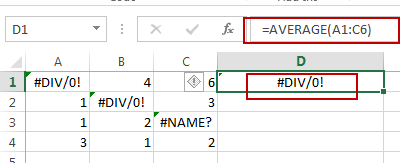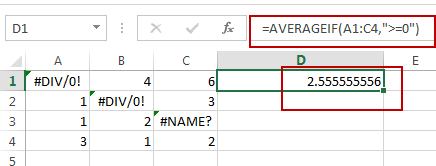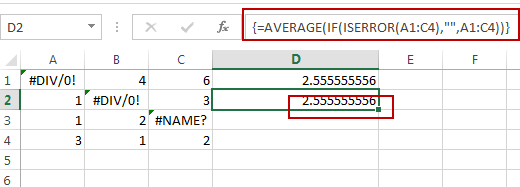# How to Ignore Error Values When Calculating the Average in Excel

This post will guide you how to ignore error values when getting average for a range of cells in Excel. How do I average a range of cells ignoring error values, such as: #div/0!.

Assuming that you have a list of range of cells that you want to calculate the average of those cells which including error values , such as: #div/0 or #NAME?, etc. When you use the Average function to calculate those cells, you will get an error result. So How to handle this in Excel.If you want to ignoring any errors that might exist in the given range, you can use AVERAGEIF function or AVERAGE function with IF function to achieve the result. Like this:

`=AVERAGEIF(A1:C4,”>=0”)`

Or

`=AVERAGE(IF(ISERROR(A1:C4),””,A1:C4))`

OR

`=AVERAGE(IF(ISNUMBER(A1:C4),A1:C4))`Note: The second and third formula need to press Ctrl + Shift +  Enter keys, as those formulas are Array Formula. A1:C4 is the data range that you wish to average. And you need to change it as you need.

Let’s see how the first formula works: the AVERAGEIF function can be used to calculate an average of numeric values with one or more criteria for a given range. And In this example, the criteria is the expression “>=0”, it will filter out all error values, and just to calculate the positive numeric values in the given range.

### Related Functions

• Excel IF function
The Excel IF function perform a logical test to return one value if the condition is TRUE and return another value if the condition is FALSE. The IF function is a build-in function in Microsoft Excel and it is categorized as a Logical Function.The syntax of the IF function is as below:= IF (condition, [true_value], [false_value])….
• Excel AVERAGE function
The Excel AVERAGE function returns the average of the numbers that you provided.The syntax of the AVERAGE function is as below:=AVERAGE (number1,[number2],…)….
• Excel AVERAGEIF function
The Excel AVERAGEAIF function returns the average of all numbers in a range of cells that meet a given criteria.The syntax of the AVERAGEIF function is as below:= AVERAGEIF (range, criteria, [average_range])….
• Excel IFERROR function
The Excel IFERROR function returns an alternate value you specify if a formula results in an error, or returns the result of the formula.The syntax of the IFERROR function is as below:= IFERROR (value, value_if_error)….
• Excel ISNUMBER function
The Excel ISNUMBER function returns TRUE if the value in a cell is a numeric value, otherwise it will return FALSE.The syntax of the ISNUMBER function is as below:= ISNUMBER (value)…